Home | | Structural Dynamics and Earthquake Engineering | Dynamic response of structures using numerical methods

# Dynamic response of structures using numerical methods

Abstract: There are two basic approaches to numerically evaluate the dynamic response. The first approach is numerical interpolation of the excitation and the second is numerical integration of the equation of motion. Both approaches are applicable to linear systems but the second approach is related to non-linear systems. In this page and next page, various numerical techniques based on interpolation, finite difference equation and assumed acceleration are employed to arrive at the dynamic response due to force and base excitation.

Dynamic response of structures using numerical methods

Abstract: There are two basic approaches to numerically evaluate the dynamic response. The first approach is numerical interpolation of the excitation and the second is numerical integration of the equation of motion. Both approaches are applicable to linear systems but the second approach is related to non-linear systems. In this chapter, various numerical techniques based on interpolation, finite difference equation and assumed acceleration are employed to arrive at the dynamic response due to force and base excitation.

Key words: time stepping, time history, central difference, explicit method, Runge-Kutta method, Newmark’s method, Wilson-θ method.

Introduction

It was clearly demonstrated in earlier chapters that analytical or closed form solution of the Duhamel integral can be quite cumbersome even for relatively simple excitation problems. Moreover, the exciting force such as an earthquake ground record cannot be expressed by a single mathematical expression, such as the analytical solution of the Duhamel integral procedure. Hence to solve practical problems, numerical evaluation techniques must be employed to arrive at the dynamic response.

To evaluate dynamic response problem there are two approaches, the first of which has two parts:

1.     Numerical interpolation of the excitation

2.     Numerical integration and Duhamel integral.

The other approach is derived by integration of the equation of motionor for the single-degree-of-freedom (SDOF) system

Both the above approaches are applicable to linear systems, but only the second approach is valid for non-linear systems.

Time stepping methods

Assume an inelastic equation to be solved asSubject to the initial condition

Usually the system is assumed to have a linear damping, but other forms of damping such as nonlinear damping should be considered. The applied force F(t) is defined at discrete time intervals and the time increment (see Fig. 7.1)may be assumed as constant, although this is not necessary. If the response is determined at the time ti, is called ith step displacement; velocity and acceleration at the ith step are denoted by u i , u˙ i , u˙˙i respectively. The displacement, velocity and acceleration are assumed to be known, satisfying Eq. 7.3 asThe third term on the left has solution gives the resisting force at time ti as fsi and for linear elastic system but would depend on the prior history of displacement and the velocity at time �'i’ if the system were inelastic. We have to discuss the numerical procedure, to determine the response quantitiesu i + 1 , u˙ i + 1 , u˙˙i +1 as i + 1th step. Similar to Eq. 7.7, at (i + 1)th step the dynamic equilibrium equation is written asif the numerical procedure is applied successively with i = 0, 1, 2,É. The time stepping procedure gives the desired response at all times with the known initial conditions u0 and u˙0 .

The time stepping procedure is not an exact procedure. The characteristics of any numerical procedure that converges to a correct answer are as follows:

Convergence - if the time step is recorded, the procedure should converge to an exact solution.

The procedure should be stable in the presence of numerical rounding errors.

The procedure should provide results close enough to the exact solution.

Types of time stepping method

There are three types of time stepping method:

1.     Methods based on the interpolation of the excitation function (See Section 7.3.1).

2.     Methods based on finite difference expressions for the velocity and acceleration (See Section 7.3.3).

3.     Methods based on assumed variation of acceleration (See Section 7.3.5).

1 Interpolation of the excitation

Duhamel integral expression for the damped and undamped SDOF system to arbitrary excitation can be solved using numerical quadrature techniques such as Simpson’s method, trapezoid method or Gauss rule. It is generally more convenient to interpolate the excitation function F(t) as (see Fig. 7.1)F(τ) is known as interpolated force and τ varies from 0 to t. Hence differential equation of noted for an undamped SDOF system becomesThe solution of Eq. 7.11 of the sum of the homogeneous and particular solutions on the time interval 0 τ ≤ ∆t. The homogeneous part of the solution is evaluated for initial condition of displacement ui and velocity u˙i at τ = 0.

The particular solution consists of two parts: (1) the response to an real step for a magnitude of Fi ; and (2) the response to a ramp function by (Fi/t)τ. Hencetion 7.12a and 7.12b are the recurrence formulae for computing displacement ui+1 and velocity u˙i +1 at time ti+1.

Recurrence formulae for a displacement and velocity under-damped system may be derived in the same manner. A simpler and more convenient representation of the recurrence formulae simplified by Eq. 7.12a and 7.12b areEquation 7.13a and 7.13b are the recurrence formulae for ρ < 1. The coefficients given in Table 7.1 depend on ωn, k and ρ and time interval t. In practice t should be sufficiently small to closely approximate the excitation force and also to render results at the required time intervals. The practice to select t T/10 where T is the natural period of the structure. This is to ensure that the important peaks of structural response are not omitted. As long as t is constant, the recurrence formulae coefficients need to be calculated once.

Example 7.1

The water tank shown in Fig.7. 2a is subjected to the blast loading illustrated in Fig 7.2b. Write a computer program in MATLAB to numerically evaluate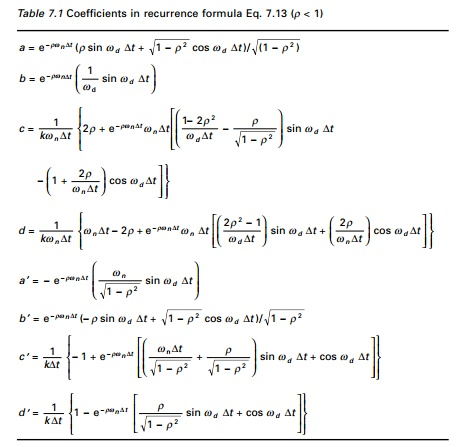the dynamic response of the tower by interpolation of the excitation. Plot the displacement u(t) and velocity u˙ (t ) response in time interval 0 t < 0.5s. Assume W = 445.5kN, k = 40913kN/m, ρ = 0.05; F0 = 445.5kN, td = 0.05s. Use the step size as 0.005s.

Calculate natural period.The constants are calculated as

a = 0.9888       a = -4.4542

b = 0.0049       b = 0.9740

c = 1.82 e-10   c = 5.4196e-08

d = 9.1305e-011   d = 5.467e-08

Using the recurrence formulae u and u˙ can be calculated as shown in Table 7.2.

The displacement time history and the velocity time history are plotted as shown in Fig. 7.3 and the program is given below.Response history for (a) displacement and (b) velocity for Example 7.1.

Program 7.1: MATLAB program for dynamic response of SDOF using recurrence formulae

%*********************************************************** % DYNAMIC RESPONSE DUE TO EXTERNAL LOAD USING WILSON RECURRENCE FORMULA

% **********************************************************

m=45412.8;

k=40913000;

wn=sqrt(k/m)

r=0.05;

u(1)=0;

v(1)=0;

tt=.50;

n=100;

n1=n+1

dt=tt/n;

td=.05;

jk=td/dt;

for m=1:n1

p(m)=0.0;

end

t=-dt

% **********************************************************

% **********************************************************

for m=1:jk+1;

t=t+dt;

p(m)=445500*(td-t)/td;

end

wd=wn*sqrt(1-r^2);

a=exp(-r*wn*dt)*(r*sin(wd*dt)/sqrt(1-r^2)+cos(wd*dt));

b=exp(-r*wn*dt)*(sin(wd*dt))/wd;

c2=((1-2*r^2)/(wd*dt)-r/sqrt(1-r^2))*sin(wd*dt)-(1+2*r/(wn*dt))*cos(wd*dt);

c=(1/k)*(2*r/(wn*dt)+exp(-r*wn*dt)*(c2));

d 2 = e x p ( - r * w n * d t ) * ( ( 2 . 0 * r ^ 2 - 1 ) / ( w d * d t ) * s i n ( w d . * d t ) + 2 . 0 * r /

(wn*dt)*cos(wd*dt));

d=(1/k)*(1-2.0*r/(wn*dt)+d2);

bd=exp(-r*wn*dt)*(cos(wd*dt)-r*sin(wd*dt)/sqrt(1-r^2));

c 1 = e x p ( - r * w n * d t ) * ( ( w n / s q r t ( 1 - r ^ 2 ) + r / ( d t * s q r t ( 1 - r^2)))*sin(wd*dt)+cos(wd*dt)/dt);

cd=(1/k)*(-1/dt+c1); d1=exp(-r*wn*dt)*(r*sin(wd*dt)/sqrt(1-r^2)+cos(wd*dt)); dd=(1/(k*dt))*(1-d1);

end

for m=1:n1 s(m)=(m-1)*dt

end figure(1);

plot(s,u,�'k’);

xlabel(�' time (t) in seconds’)

ylabel(�' Response displacement u in m’) title(�' dynamic response’)

figure(2);

plot(s,v,�'k’);

xlabel(�' time (t) in seconds’)

ylabel(�' Response velocity v in m/sec’) title(�' dynamic response’)

3     Direct integration of equation of motion

In direct integration, the equation of motion is integrated using a step-by-step procedure. It has two fundamental concepts: (1) the equation of motion is satisfied at only discrete time intervals t and (2) for any time t, the variation of displacements, velocity and acceleration with each time interval t is assumed.

Consider the SDOF systemIt is assumed that displacement velocity and acceleration at time t = 0 are given as u0, u˙ 0, u˙˙0 respectively. Algorithms can be derived to calculate the solution at some time t + t based upon the solution at time ‘t’. Several commonly based direct integration methods are presented below.

Central difference method

This method is based on finite difference approximation of the time derivations of displacements (velocity and acceleration). Taking a constant time step tThe above method uses equilibrium condition at time i but does not satisfy equilibrium condition at i + 1.

In Eq. 7.17b it is observed that known displacements of ui, ui-1 are used to interpolate ui+1. Such methods are called explicit methods. In Eq. 7.17b, it is observed that known displacements of ui, ui-1 are required to determine ui+1. Thus u0, u-1 are required to determine u1.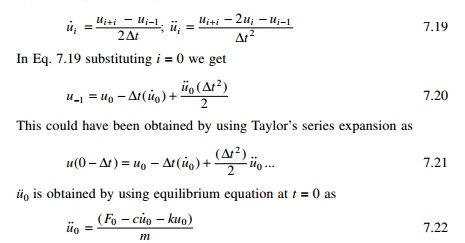This is not a constraint for SDOF systems because a much smaller time step is chosen for better accuracy. The steps taken in the central difference method are given in Table 7.3.

Example 7.2

A single story shear frame shown in Fig. 7.4a is subjected to arbitrary excitation force specified in Fig. 7.4b. The rigid girder supports a load of 25.57kN/m.Assume the columns bend about their major axis and neglect their mass, and assuming damping factor of ρ = 0.02 for steel structures, E = 200GPa. Write a computer program for the central difference method to evaluate dynamic response for the frame. Plot displacement u(t), velocity v(t) and acceleration a(t) in the interval 0 t 5s.

Solution

(a)  The total load on the beam = 25.57 �- 10 = 255.7kNTable 7.4 gives the displacement, velocity and acceleration up to 1s.

The displacement, velocity and acceleration response are shown in Fig. 7.5. The computer program in MATLAB is given below.

Program 7.2: MATLAB program for dynamic response of SDOF using central difference method

%**********************************************************

%DYNAMIC RESPONSE USING CENTRAL DIFFERENCE METHOD

%**********************************************************

ma=26065;

k=2369904.0;

wn=sqrt(k/ma)

r=0.02;

c=2.0*r*sqrt(k*ma)

u(1)=0;

v(1)=0;

tt=5;

n=100;n1=n+1

dt=tt/n;

td=.9;

jk=td/dt;

%*********************************************************** for m=1:n1

p(m)=0.0; end

t=-dt

for m=1:8; t=t+dt; p(m)=20000;

end p(9)=16000.0 for m=10:12 t=t+dt p(m)=12000.0 end

for m=13:19 t=t+dt

p(m)=12000.0*(1-(t-0.6)/.3) end an(1)=(p(1)-c*v(1)-k*u(1))/ma up=u(1)-dt*v(1)+dt*dt*an(1)/2 kh=ma/(dt*dt)+c/(2.0*dt) a=ma/(dt*dt)-c/(2.0*dt) b=k-2.0*ma/(dt*dt) f(1)=p(1)-a*up-b*u(1) u(2)=f(1)/kh

for m=2:n1 f(m)=p(m)-a*u(m-1)-b*u(m) u(m+1)=f(m)/kh

end v(1)=(u(2)-up)/(2.0*dt) for m=2:n1

v(m)=(u(m+1)-u(m-1))/(2.0*dt) an(m)=(u(m+1)-2.0*u(m)+u(m-1))/(dt*dt) end

n1p=n1+1 for m=1:n1p

s(m)=(m-1)*dt end

for m=1:n1 x(m)=(m-1)*dt end

figure(1);

plot(s,u,’k’);

xlabel(‘ time (t) in seconds’)

ylabel(‘ Response displacement u in m’) title(‘ dynamic response’)

figure(2);

plot(x,v,’k’);

xlabel(‘ time (t) in seconds’)

ylabel(‘ Response velocity v in m/sec’) title(‘ dynamic response’)

figure(3);

plot(x,an,’k’);

xlabel(‘ time (t) in seconds’)

ylabel(‘ Response acceleration a in m/sq.sec’) title(‘ dynamic response’)

4.Single step methods

Runge�'Kutta method

These methods are classified as single step since they require knowledge of only xi to determine xi+1. Hence the methods are called self-starting as they require no special starting procedure unlike the central difference method. The Runge-Kutta method of order 4 is usually applied in practice. Consider the differential equation of motion for a single degree of freedom asEquations 7.26a and 7.26b represent an averaging of the velocity and acceleration by Simpson’s rule within the time interval t. The fourth order Runge-Kutta method is summarized in Table 7.5.Example 7.3

A steel water tank shown in Fig. 7.6a is analysed as an SDOF system having a mass on top of cantilever which acts as a spring and dashpot for damping. A blast load of P(t) is applied as shown in Fig. 7.6b. The values of the force are given in Table 7.6. Draw displacement, velocity and acceleration responses up to 0.5s. The damping for steel may be assumed to be 2% of critical damping.Solution

Given

W = 133.5kNFigure 7.7 shows the displacement, velocity and acceleration response for the tank. The values are given in Table 7.7 and the program is given below.

%******************************************************************** % DYNAMIC RESPONSE DUE TO EXTERNAL LOADING RUNGE KUTTA METHOD %******************************************************************** ma=13608.5;

k=17500000;

wn=sqrt(k/ma)

r=0.02;

c=2.0*r*sqrt(k*ma)

u(1)=0;

v(1)=0;

tt=.5;

n=50;

n1=n+1

dt=tt/n;

td=.1;

jk=td/dt;p(m)=0.0; end p(2)=267000.0 p(3)=445000.0 p(4)=364000.0 p(5)=284000.0 p(6)=213000.0 p(7)=142000.0 p(8)=89000.0

p(9)=53400.0

p(10)=26700.0 an(1)=(p(1)-c*v(1)-k*u(1))/ma t=0.0

for i=2:n1 ui=u(i-1) vi=v(i-1) ai=an(i-1) d(1)=vi q(1)=ai for j=2:3 l=0.5

x=ui+l*dt*d(j-1) d(j)=vi+l*dt*q(j-1) q(j)=(p(i)-c*d(j)-k*x)/ma end

j=4

l=1 x=ui+l*dt*d(j-1) d(j)=vi+l*dt*q(j-1)

q(j)=(p(i)-c*d(j)-k*x)/ma u(i)=u(i-1)+dt*(d(1)+2.0*d(2)+2.0*d(3)+d(4))/6.0 v(i)=v(i-1)+dt*(q(1)+2.0*q(2)+2.0*q(3)+q(4))/6.0 an(i)=(p(i)-c*v(i)-k*u(i))/ma

end

for i=1:n1 s(i)=(i-1)*dt end figure(1);

plot(s,u,’k’);

xlabel(‘ time (t) in seconds’)

ylabel(‘ Response displacement u in m’) title(‘ dynamic response’)

figure(2);

plot(s,v,’k’);

xlabel(‘ time (t) in seconds’)

ylabel(‘ Response velocity v in m/sec’) title(‘ dynamic response’)

figure(3);

plot(s,an,’k’);

xlabel(‘ time (t) in seconds’)

ylabel(‘ Response acceleration a in m/sec’) title(‘ dynamic response’)

figure(4);

plot(s,p,’k’)

xlabel(‘ time (t) in seconds’) ylabel(‘ force in Newtons’) title(‘ Excitation Force’)

7.3.5     Assumed acceleration methods

Average acceleration method

It is assumed that with a small increment of time t, the acceleration is the average value of the acceleration at the beginning of the interval u˙˙i and the acceleration at the end of the interval u˙˙i +1 as illustrated in Fig. 7.8. Hence acceleration at the some time τ between ti and ti+1 can be expressed as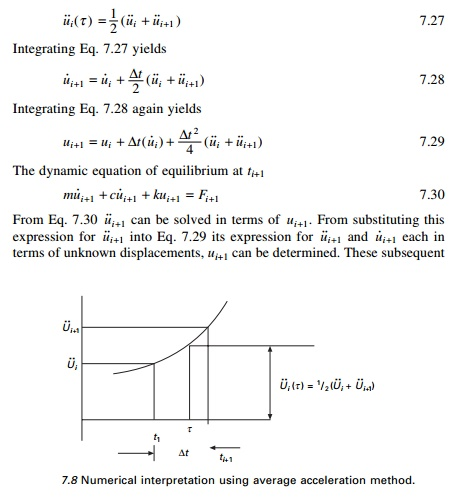A computational algorithm can be developed in terms of incremental quantities for applied load Fi for displacement ui for velocity u˙i and for acceleration u˙˙i quantities asEven though this kind of incremental form is not necessary for linear systems, it is required for nonlinear systems and with non-proportional damping which we will see in a later chapter. Table 7.8 gives a step-by-step solution using the average acceleration method (incremental form).

Table 7.8 Average acceleration methodIt can be proved that the average acceleration method or constant acceleration method just discussed is equivalent to the trapezoidal rule. It is also a special form of the Newmark method which will be discussed later.

Example 7.4

The shear frame shown in Fig. 7.9a is subjected to the exponential pulse force shown in Fig 7.9b. Write a computer program for the average acceleration method (incremental formulation) to evaluate the dynamic response of the frame. Plot the time histories for displacement u(t), velocity u˙ (t ) and acceleration u˙˙(t ) in the time interval 0-3s. Assume E = 200 GPa, W = 1079.1kN, ρ = 0.07, F0 = 450kN and td = 0.75s and use a time step t of 0.01s.

Solution

Given

I for ISWB 600 @1337 = 106 198.5e4mm4

Mass = m = 110 000 kg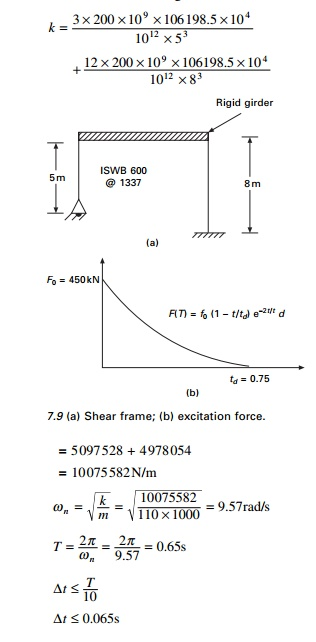t 0.065s

We use t = 0.01s.

The displacement velocity and acceleration response are shown in Fig. 7.10 and the values are given in Table 7.9. The program using MATLAB for constant acceleration method is given below.

Program 7.4: MATLAB program for dynamic response by constant acceleration method

% Response by constant acceleration method. ma=110000;k=10075582;

wn=sqrt(k/ma)

r=0.07;

c=2.0*r*sqrt(k*ma)

u(1)=0;

v(1)=0;

tt=3;

n=300;

n1=n+1

dt=tt/n;

td=.75;

jk=td/dt; for m=1:n1

p(m)=0.0; end jk1=jk+1 for n=1:jk1 t=(n-1)*dt

p(n)=450000*(1-t/td)*exp(-2.0*t/td) end

an(1)=(p(1)-c*v(1)-k*u(1))/ma kh=k+4.0*ma/(dt*dt)+2.0*c/dt for i=1:n1

s(i)=(i-1)*dt end

for i=2:n1 ww=p(i)-p(i-1)+(4.0*ma/dt+2.0*c)*v(i-1)+2.0*ma*an(i-1) xx=ww/kh

yy=(2/dt)*xx-2.0*v(i-1) zz=(4.0/(dt*dt))*(xx-dt*v(i-1))-2.0*an(i-1) u(i)=u(i-1)+xx

v(i)=v(i-1)+yy an(i)=an(i-1)+zz end

figure(1);

plot(s,u);

xlabel(‘ time (t) in seconds’)

ylabel(‘ Response displacement u in m’) title(‘ dynamic response’)

figure(2);

plot(s,v);

xlabel(‘ time (t) in seconds’)

ylabel(‘ Response velocity v in m/sec’) title(‘ dynamic response’)

figure(3);

plot(s,an);

xlabel(‘ time (t) in seconds’)

ylabel(‘ Response acceleration a in m/sec’) title(‘ dynamic response’)

figure(4);

plot(s,p)

xlabel(‘ time (t) in seconds’) ylabel(‘ force in Newtons’) title(‘ Excitation Force’)

6.Assumed acceleration method (linear variation)

Linear acceleration method

In this method a linear variation of acceleration from time ti to ti+1 is assumed as illustrated in Fig 7.11. Let τ denote the time within the interval ti and ti+1 such that 0 τ ≤ ∆t (see Fig. 7.11). Then acceleration at time τ is expressed asAfter determining ui+1 Eq. 7.45a and 7.45b may be used to determine velocity u˙i+1 and acceleration u˙˙i+1 . The above algorithm is for total formulation. However this algorithm can be modified for incremental formulation as shown in Table 7.10.

The displacement velocity and acceleration are almost the same as in the average acceleration method and the response curve is exactly the same as in Fig. 7.10. The program for linear acceleration method in MATLAB is given below.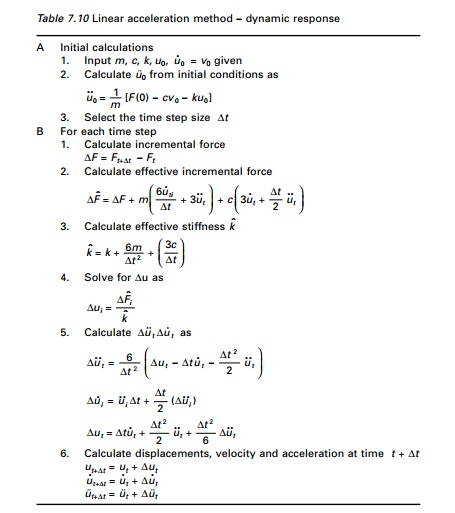Program 7.5: MATLAB program for dynamic response by linear acceleration method% Linear Acceleration Method. %******************************* ma=110000;

k=10075582;

wn=sqrt(k/ma)

r=0.07;

c=2.0*r*sqrt(k*ma)

u(1)=0;

v(1)=0;

tt=3;

n=300;

n1=n+1

dt=tt/n;

td=.75;

jk=td/dt; for m=1:n1

p(m)=0.0; end jk1=jk+1 for n=1:jk1 t=(n-1)*dt

p(n)=450000*(1-t/td)*exp(-2.0*t/td) end

an(1)=(p(1)-c*v(1)-k*u(1))/ma kh=k+6.0*ma/(dt*dt)+3.0*c/dt for i=1:n1

s(i)=(i-1)*dt end

for i=2:n1 ww=p(i)-p(i-1)+ma*(6.0*v(i-1)/dt+3.0*an(i-1))+c*(3.0*v(i-1)+dt*an(i-1)/2) xx=ww/kh

zz=(6.0/(dt*dt))*(xx-dt*v(i-1)-dt*dt*an(i-1)/2) yy=dt*an(i-1)+dt*zz/2.0 vv=v(i-1)*dt+(dt*dt)*(3.0*an(i-1)+zz)/6.0 v(i)=v(i-1)+yy

an(i)=an(i-1)+zz u(i)=u(i-1)+vv end

figure(1);

plot(s,u);

xlabel(‘ time (t) in seconds’)

ylabel(‘ Response displacement u in m’) title(‘ dynamic response’)

figure(2);

plot(s,v);

xlabel(‘ time (t) in seconds’)

ylabel(‘ Response velocity v in m/sec’) title(‘ dynamic response’)

figure(3);

plot(s,an);

xlabel(‘ time (t) in seconds’)

ylabel(‘ Response acceleration a in m/sec’)

title(‘ dynamic response’) figure(4);

plot(s,p)

xlabel(‘ time (t) in seconds’) ylabel(‘ force in Newtons’) title(‘ Excitation Force’)

7.Stepping methods

Newmark’s method

In 1959, N.M. Newmark developed a time stepping method based on the following equations:The parameter β and γ define the variation of acceleration over a time step and determine the stability and accuracy characteristics of the method. Usually

γ is selected as 1/2 and  16 β 14  is satisfactory from all points of view,

including accuracy. Equation 7.48 combined with an equilibrium equation at the end of the time step provides the basis of computing u i + 1 , u˙ i + 1 , u˙˙i +1 knowing the values of ui , u˙ i , u˙˙i .

For linear systems as the ones discussed in the chapter there is no iteration needed. Newmark’s method for linear systems is given in Table 7.11. It is proved that Newmark’s method is stable ifThe above proves that the average acceleration method is stable for any t, no matter how large; however, it is accurate only if t is small enough. For the linear acceleration method γ = 1/2, β = 1/6 and that is stable ifTo get an accurate estimate a shorter time step must be used:

The program for Newark’s method’s given in below. Example 7.4 is solved using Newark’s method (γ = 1/2, β = 1/6) - linear acceleration method - and we get displacement, velocity and acceleration time response as shown in Fig 7.10.Program 7.6: MATLAB program for Nemark’s method for linear systems

%***********************************************************

%     NEWMARK’S METHOD FOR AVERAGE OR LINEAR ACCELERATION METHODS

%BETA=0.25 .... AVERAGE ACCELERATION METHOD

%BETA = 0.167 .... LINEAR ACCELERATION METHOD %*********************************************************** ma=110000;

k=10075582;

wn=sqrt(k/ma)

gamma=0.5

beta=0.25

r=0.07;

c=2.0*r*sqrt(k*ma)

u(1)=0;

v(1)=0;

tt=3.0;

n=300;

n1=n+1

dt=tt/n;

td=.75;

a=ma/(beta*dt)+gamma*c/beta b=ma/(2.0*beta)+dt*c*(gamma/(2.0*beta)-1) jk=td/dt;

%*********************************************************** % THIS IS WHERE LOAD IS DEFINED %*********************************************************** for m=1:n1

p(m)=0.0; end jk1=jk+1 for n=1:jk1 t=(n-1)*dt

p(n)=450000*(1-t/td)*exp(-2.0*t/td) end

an(1)=(p(1)-c*v(1)-k*u(1))/ma kh=k+ma/(beta*dt*dt)+gamma*c/(beta*dt) for i=1:n1

s(i)=(i-1)*dt end

for i=2:n1 ww=p(i)-p(i-1)+a*v(i-1)+b*an(i-1) xx=ww/kh

zz=xx/(beta*dt*dt)-v(i-1)/(beta*dt)-an(i-1)/(2.0*beta) yy=(gamma*xx/(beta*dt)-gamma*v(i-1)/beta+dt*(1-gamma/(2.0*beta))*an(i-1))

v(i)=v(i-1)+yy an(i)=an(i-1)+zz

vv=dt*v(i-1)+dt*dt*(3.0*an(i-1)+zz)/6.0 u(i)=u(i-1)+vv

end figure(1);

plot(s,u,’K’);

xlabel(‘ time (t) in seconds’)

ylabel(‘ Response displacement u in m’) title(‘ dynamic response’)

figure(2);

plot(s,v,’K’);

xlabel(‘ time (t) in seconds’)

ylabel(‘ Response velocity v in m/sec’) title(‘ dynamic response’)

figure(3);

plot(s,an,’K’);

xlabel(‘ time (t) in seconds’)

ylabel(‘ Response acceleration a in m/sec’) title(‘ dynamic response’)

figure(4);

plot(s,p,’K’)

xlabel(‘ time (t) in seconds’) ylabel(‘ force in Newtons’) title(‘ Excitation Force’)

7.3.8     Conditionally stable method

Wilson-θ method

A method developed by E L Wilson is a modification of the conditionally stable linear acceleration method that makes it unconditionally stable. This is based on the assumption that acceleration varies linearly over an extended time step δt = θt as shown in Fig. 7.12. The accuracy and stability properties of the method depend on the value θ which is always greater than 1.

The numerical procedure is derived in a similar line of linear acceleration methods. Everything described in this chapter will be useful to a multiple-degrees-of-freedom (MDOF) non-linear system with non-proportional damping. The incremental velocity and incremental acceleration can be given asand the incremental velocity and displacement are determined from Eq. 7.52a, 7.52b. For the MDOF system δui is determined using a tangent stiffness matrix and an iterative procedure. Table 7.12 gives the algorithm for the Wilson-θ method.As discussed earlier, the value of θ governs the stability characteristics of the Wilson-θ method. If θ = 1 this method is the linear acceleration method, which is stable for t< 0.551 Tn when Tn is the shortest natural period of time. If θ 1.37 the Wilson-θ method is unconditionally stable, making it suitable for direct solution ofIt is proved by Wilson that θ = 1.42 gives optimal accuracy.

The computer program in MATLAB for Wilson-θ method for Example 7.4 is given below and we get the displacement. The velocity and acceleration response are the same as Fig. 7.10.

Program 7.7: MATLAB program for dynamic response by Wilson-θ Method

% Wilson theta method %********************** ma=110000;

k=10075582;

wn=sqrt(k/ma)

theta=1.42

r=0.07;

c=2.0*r*sqrt(k*ma)

u(1)=0;

v(1)=0;

tt=3.0;

n=300;

n1=n+1

dt=tt/n;

td=.75;

jk=td/dt; for m=1:n1

p(m)=0.0;

end;

jk1=jk+1; for n=1:jk1; t=(n-1)*dt;

p(n)=450000*(1-t/td)*exp(-2.0*t/td); end; an(1)=(p(1)-c*v(1)-k*u(1))/ma;

kh=k+3.0*c/(theta*dt)+6.0*ma/(theta*dt)^2;

a=6.0*ma/(theta*dt)+3.0*c;

b=3.0*ma+theta*dt*c/2.0; for i=1:n1;

s(i)=(i-1)*dt; end;

for i=2:n1; ww=(p(i)-p(i-1))*theta+a*v(i-1)+b*an(i-1); xx=ww/kh;

zz=(6.0*xx/((theta*dt)^2)-6.0*v(i-1)/(theta*dt)-3.0*an(i-1))/theta; yy=dt*an(i-1)+dt*zz/2.0;

v(i)=v(i-1)+yy; an(i)=an(i-1)+zz;

vv=dt*v(i-1)+dt*dt*(3.0*an(i-1)+zz)/6.0; u(i)=u(i-1)+vv;

end;

figure(1);

plot(s,u);

xlabel(‘ time (t) in seconds’)

ylabel(‘ Response displacement u in m’) title(‘ dynamic response’)

figure(2);

plot(s,v);

xlabel(‘ time (t) in seconds’)

ylabel(‘ Response velocity v in m/sec’) title(‘ dynamic response’)

figure(3);

plot(s,an);

xlabel(‘ time (t) in seconds’)

ylabel(‘ Response acceleration a in m/sec’) title(‘ dynamic response’)

figure(4);

plot(s,p)

xlabel(‘ time (t) in seconds’) ylabel(‘ force in Newtons’) title(‘ Excitation Force’)

Study Material, Lecturing Notes, Assignment, Reference, Wiki description explanation, brief detail
: Dynamic response of structures using numerical methods |